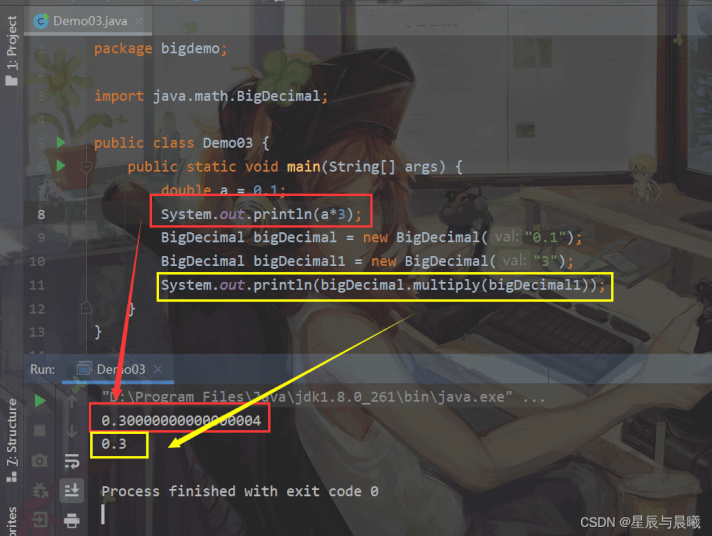﻿ Java你不了解的大数型BigInteger与BigDecimal类_java_脚本之家
java# Java你不了解的大数型BigInteger与BigDecimal类

## BigInteger类

BigInteger类支持的数字可以说是无限大的，且支持任意精度的整数，也就是说他可以准确的表示任意数值而不会产生丢失的。

equals方法。进行比较

```import java.math.BigInteger;
public class Demo01 {
public static void main(String[] args) {
BigInteger bigInteger1 = new BigInteger("2222222222222222222222222222222");
BigInteger bigInteger2 = new BigInteger("1111111111111111111111111111111");
System.out.println("bigInteger1大数为：" + bigInteger1);
System.out.println("bigInteger2大数为：" + bigInteger2);
System.out.println("两个大数相减：" + bigInteger1.subtract(bigInteger2));//减
System.out.println("两个大数相乘：" + bigInteger1.multiply(bigInteger2));//乘
System.out.println("两个大数相除：" + bigInteger1.divide(bigInteger2));//除
System.out.println("两个大数相比：" + bigInteger1.equals(bigInteger2));//equals进行比较
}
}
```## BigDecimal类

```import java.math.BigDecimal;
public class Demo02 {
public static void main(String[] args) {
BigDecimal bigDecimal1 = new BigDecimal("12232423432432.53241234324");
BigDecimal bigDecimal2 = new BigDecimal("2.0");
System.out.println("bigDecimal1值： " + bigDecimal1);
System.out.println("bigDecimal2值： " + bigDecimal2);
System.out.println("减：" + bigDecimal1.subtract(bigDecimal2));
System.out.println("乘：" + bigDecimal1.multiply(bigDecimal2));
System.out.println("除：" + bigDecimal1.divide(bigDecimal2));
}
}
```## BigDecimal类当中除法除不尽问题public BigDecimal divide(BigDecimal divisor, int scale, int roundingMode)

divisor - 除数。

scale - 小数点后保留几位

roundingMode - 选择舍入模式ROUND_CEILING向正无穷取数
ROUND_DOWN向0取数
ROUND_FLOOR向负无穷取数
ROUND_HALF_DOWN四舍五入遇到 .5向下取数
ROUND_HALF_UP四舍五入遇到 .5向上取数
ROUND_HALF_EVEN四舍五入遇到5看前面的数，大于5向上，小于5向下，等于5再向前看。